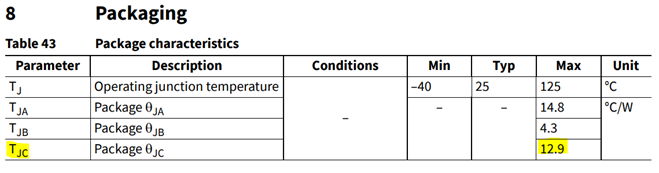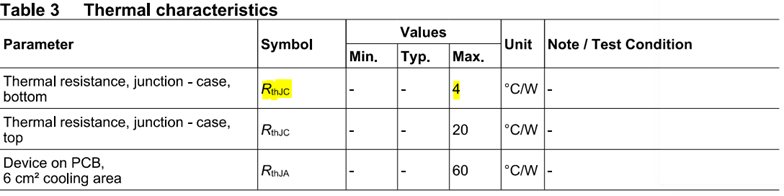# Calculating the case temperature (Tc) of MOSFET and controller in CCG7DC – KBA236784ModeratorModerator# Calculating the case temperature (Tc) of MOSFET and controller in CCG7DC – KBA236784

To calculate the device's maximum case temperature, use the following formula:

Tcase-max = Tjunction-max-(θjunction-to-case×Pdissipated)

Where,

• Tcase-max : Maximum case temperature
• Tjunction-max : Maximum junction temperature
• θjunction-to-case : Thermal resistance of junction to case
• Pdissipated : Power dissipation

Case1: Case temperature (Tc) of CCG7DC controller

1. The operating temperature of the CCG7DC is between -40 and 105.
2. If extended till 125, it may shorten the useful life of the device.
3. Usage above the absolute maximum conditions, i.e., above 125 may cause permanent damage to the device.

The CCG7DC thermal resistance of the case is 12.9/W. See Figure 1 for datasheet reference.Figure 1 Example datasheet

In Figure 1Tcase-max = Tjunction-max -12.9*PD
–40
<Tj  < 125

Case 2:
Case temperature (Tc) of MOSFET

BSZ063N04LS6 is considered for analysis since it is used in the 45W USB-PD Dual C Power Adapter Solution with PAG1P-A3/PAG1S-A1+CCG7D-C controllers.

The operating temperature of the MOSFET is between -55 and 175 ℃.

The MOSFET thermal resistance of the case is 4/W. See Figure 2 for datasheet reference.Figure 2 Example datasheet

In Figure 2Tcase-max = Tjunction-max -4*PD
–55
<Tj  < 175

Note: Higher the Power Dissipation (Pd), lower the maximum case temperature.

Example: Tc of MOSFET

Considering that Tj   is 125 and 20W of power is dissipated, the case temperature under the given conditions is as follows:

Tcase-max = Tjunction-max -4*PD
= 125 – 4*20
= 45℃

102 Views
Contributors